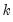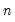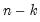makepanpcomp
Save the scores from a principal components analysis of a panel series.
Syntax
series_name.makepanpcomp(options) output_list
where the output_list is a list of names identifying the saved components. EViews will save the firstcomponents corresponding to theelements in output_list, up to the total number of series in the group.
Options

 scale=arg (default=“normload”) Diagonal matrix scaling of the loadings and the scores: normalize loadings (“normload”), normalize scores (“normscores”), symmetric weighting (“symmetric”), user-specified (arg=number). cpnorm Compute the normalization for the score so that cross-products match the target (by default, EViews chooses a normalization scale so that the moments of the scores match the target). eigval=vec_name Specify name of vector to hold the saved the eigenvalues in workfile. eigvec=mat_name Specify name of matrix to hold the save the eigenvectors in workfile. prompt Force the dialog to appear from within a program.
Covariance Options

 period Compute period (within cross-section) panel covariances and related statistics. The default is to compute contemporaneous (between cross-section) measures. cov=arg (default=“corr”) Covariance calculation method: ordinary (Pearson product moment) covariance (“cov”), ordinary correlation (“corr”), Spearman rank covariance (“rcov”), Spearman rank correlation (“rcorr”), uncentered ordinary correlation (“ucorr”). Note that Kendall’s tau measures are not valid methods. pairwise Compute using pairwise deletion of observations with missing cases (pairwise samples). df Compute covariances with a degree-of-freedom correction accounting for the estimation of the mean (for centered specifications). The default behavior in these cases is to perform no adjustment (e.g. – compute sample covariance dividing byrather than).
Examples
ser1.makepanpcomp(eigval=v1, eigvec=m1) comp1 comp2 comp3
saves the first three principal components (in normalized loadings form) of the panel series SER1 to the workfile. The components will have variances that are proportional to the eigenvalues of the contemporaneous correlation matrix. In addition, the vector V1 and matrix M1 will contain the eigenvectors and eigenvalues of the decomposition.
smpl 1990 2010
ser.makepanpcomp(period, cov=rcorr, scale=normscore) comp1
saves the first principal component of the period (within cross-section) Spearman rank correlations. The scores will be normalized so that the variances of the scores are equal to 1.
Cross-references
See “Saving Component Scores” and “Panel Principal Components” for further discussion.
To display the results of the panel principal components decomposition, see Series::panpcomp.